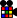# Difference between revisions of "Template:A-PLL(a)"

### A Permutation : a

 Name: A-PLL a Used in: CPLL, PLL, L3C, L4C, ZBLL, BLD Optimal moves: 9 HTMPLL R' F R' B B R F' R' B B R R U'PLL (x') R' D R' U2 R D' R' U2 R2 (x)PLL (x) R' U R' D2 R U' R' D2 R2 (x')PLL l' U R' D2 R U' R' D2 R2 (x')PLL R' F R' B2 R F' R' B2 R2PLL l' U R' (z) r2 U R' U' r2 (z) L2' (x')PLL (y x') R2 u2 r' F' r u2 R' U l'PLL (y' x) L2 D2 L' U' L D2 L' U L' (x')PLL (y2 z) F2 R U2 R' U2 F2 L' U2 L U2 (z')PLL (y z') U2 R U2 R' F2 U2 L' U2 L F2 (z)PLL R' U2 R2 U' L' U R' U' L U R' U2 RPLL R U' L' U' L2 U' R2 U L2 U' R2 U2 L U R'PLL (y') R U R' F' r U R' U' r' F R2 U' R'PLL (y') r U r' U' r' F r2 U' r' U' r U r' F'